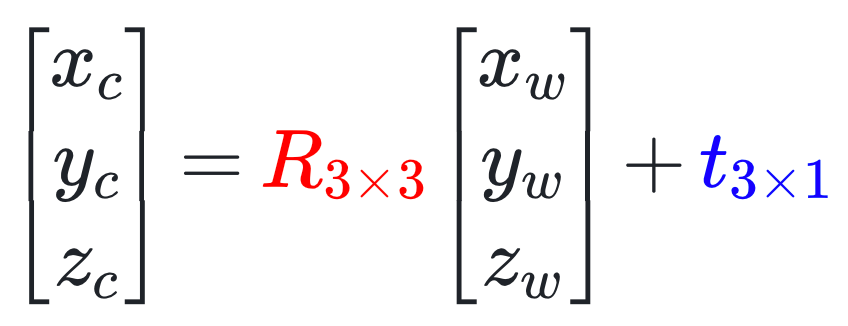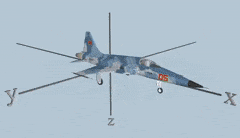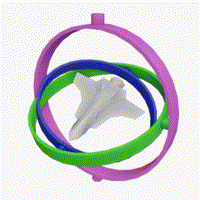top of page

# Camera & LiDAR Sensor Fusion Key Concept: Extrinsic Parameters

## 1. Background

You might have had this experience in school or everyday life: a teacher says "left" first, but then immediately corrects themselves and says "right." Maybe you've had similar moments when teaching someone else.Teacher and Students in the opposite direction

Why does this happen? It's because when facing each other, the teacher's left and right are opposite to the student's left and right. So, as mentioned in the previous article, the coordinate system within a LiDAR's "eyes" and the coordinate system within a camera's "eyes" are two different systems. Their x, y, and z axes are not congruent, just like how two people's left and right are different.

How do we solve this conflict? It's not too difficult – if a teacher wants to experience the student's perspective, they simply need to walk to their position, turn around, and face the blackboard.Teacher and Student in the same direction

This example reveals the transformation that a coordinate needs to undergo from the radar coordinate system to the camera coordinate system: translation and rotation. The parameters related to these two transformations are called extrinsic parameters.

## 2. Overview of Extrinsic Parameters

The definition of intrinsic parameters is very clear, and their representation is relatively uniform, namely two scale-related parameters fx, fy, and two translation-related parameters cx, cy, totaling four parameters.

Extrinsic parameters are different – their representation varies, and different people have different definitions. This leads to the complexity of handling extrinsic parameters.

First, some people are used to using extrinsic parameters to describe the change from the radar coordinate system to the camera coordinate system, while others prefer to use extrinsic parameters to describe the change from the camera coordinate system to the radar coordinate system (this article adopts the former).

Second, unlike the intrinsic parameters' unified use of diagonal matrices to represent scaling transformations, rotation matrices, rotation vectors/MRPs, Euler angles, and quaternions can all represent rotation transformations in the extrinsic parameter part.Extrinsic parameters consist of R and T

## 3. Various Forms of Extrinsic Parameters

### 3.1 Extrinsic Matrix

Let's start with the familiar matrix and introduce them one by one.

Rotation transformation is a linear transformation that can be represented by matrix multiplication; translation transformation, as mentioned in the previous article, is represented by addition. The complete transformation is expressed as follows:In the equation above, R3x3 represents a 3x3 rotation matrix, and t3x1 represents a 3x1 translation vector.

Upon closer inspection, isn't this the affine transformation mentioned in the previous article? Therefore, it can also be represented as a single matrix multiplication after increasing its dimension:The 4x4 matrix in the equation above is the extrinsic matrix. Since the last row consists of fixed 0s and 1s, it can also be represented like this:It can be simplified as:Rotation matrices are quick and easy to compute, but they don't provide an intuitive understanding of the rotation angle and direction. Let's dive into various ways to represent rotation transformations.

### 3.2 Rotation Vector

Imagine you're trying to describe the location of the nearest museum to a friend: you might say, "It's 1 kilometer northeast of my house." You're directly using direction and distance to convey the relative position of two locations.See the red line

The rotation vector works similarly—it's a concise three-dimensional vectorThe unit vector represents the rotation axis:and the magnitude represents the angle:This means that the rotation occurs around the r axis by θ degrees.

### 3.3 MRPs (Modified Rodrigues Parameters)

MRPs are another three-dimensional vector. Essentially, they're the same as rotation vectors, using one axis and one angle to represent rotation. The only difference is the relationship between magnitude and the angle:For example, if we want to represent a 180° rotation, the vector's magnitude is 1.

### 3.4 Euler Angles

Continuing our museum example, sometimes the straight-line distance is blocked by buildings. So, you might say, "Walk 0.7 kilometers east, then 0.7 kilometers north, and you're there."See the blue line

Euler angles work similarly, using three rotations around three axes to represent one overall rotation. This is the most intuitive way to represent rotation. Euler angles can be static or dynamic, referring to whether the world coordinate system (xyz) or the object's coordinate system (XYZ) is used as the reference. Let's use dynamic Euler angles as an example:In the animation above, Ψ is the yaw angle, θ is the pitch angle, and Φ is the roll angle. The rotation can be described as follows: first, rotate around the plane's Z-axis by Ψ degrees, then around the rotated plane's Y-axis by θ degrees, and finally around the rotated plane's X-axis by Φ degrees. Formally, Euler angles are also a three-dimensional vector.

However, Euler angles involve rotation order. For instance, rotating around the x-axis, then the y-axis generally produces different results than rotating around the y-axis followed by the x-axis. When using Euler angles, you must specify the order (e.g., 'ZYX' as shown above).

### 3.5 Quaternions

#### 3.5.1 Quaternion Definition

While Euler angles are easy to understand, they can cause gimbal lock issues, which can not be ignored in applications like 3D modeling.As shown above, when the green and purple circles align, rotating the blue or purple circles results in the same rotation, leading to a loss of degrees of freedom.

Quaternions are an alternative for representing rotation. They are more abstract and harder to understand than Euler angles but offer many advantageous properties. A quaternion is defined as: q=iqx+jqy+kqz+qw, where i^2=j^2=k^2=-1, jk=-kj=i, ki=-ik=j, ij=-ji=k, qw is the real part, iqx+jqy+kqz is the imaginary part, and i, j, k are imaginary units.

#### 3.5.2 Quaternion Degrees of Freedom

Quaternion multiplication can be broken down as follows:where the left side (q1/||q1||) represents the rotation, and the right side ||q1||·q2 represents scaling.

You might have noticed that since we're only discussing 3D rotation, the quaternion's magnitude doesn't matter. Therefore, we use unit quaternions when representing rotation, which restrictsand explains why we need a four-element quaternion to represent the three degrees of freedom in 3D rotation.

#### 3.5.3 Simplified Quaternion Understanding

For easier understanding, we can view quaternions in a simpler way (omitting imaginary units):Where rxry and rz are the components of the unit rotation axis vector, and θ is the rotation angle. This representation is similar to the rotation vector, but with an additional scalar component .